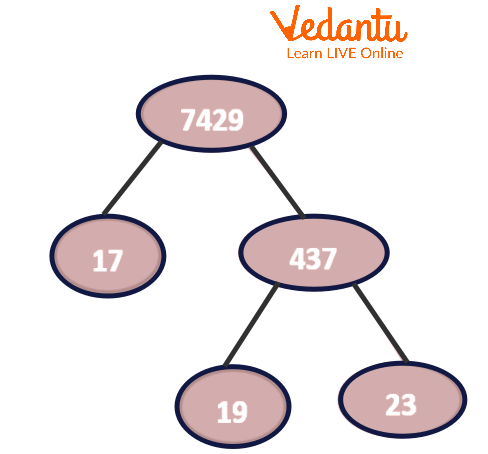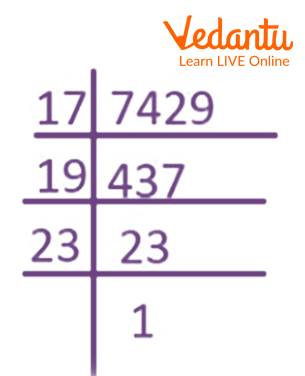Courses
Courses for Kids
Free study material
Free LIVE classes
More

# Finding the Factors of 7429LIVE
Join Vedantu’s FREE Mastercalss

## What are the Factors of 7429?

Factors of a number are integers that when divided by the given number leave remainder as 0. The integers that can divide 7429 evenly i.e., without any remainder are known as the factors of 7429. Finding the factors for bigger numbers like 7429, however, is challenging since it might be hard to determine the numbers that divide it exactly. We can use both the division and factor tree methods to identify the factors.

## How to Find the Factors of 7429?

To understand how to find the factors of 7429, follow the steps provided below.

• Step 1: Find the two integers that when multiplied together result in 7429, for example, $17\; \times \;437\; = \;7429$.

• Step 2: We are aware that the number 17 is a prime number. Therefore, we are unable to factor it further.

• Step 3: Let's now try with various multiplications of integers that result in the number 7429, such as

$19\; \times \;391\; = \;7429$

$23\; \times \;323\; = \;7429$

Therefore, 1, 17, 19, 23, 323, 391, 437, and 7429 are the factors of 7429.

## Prime Factors of 7429

In order to find the prime factors of 7429, one can also use the factor tree method. A factor tree involves creating branches of prime numbers that are factors of the given number. The end numbers in the factor tree denote the prime factors of the given number.Factor Tree of 7429

Due to its composite nature, the number 7429 will have prime factors. The factor tree of 7429 as represented in the above image shows that 7429 has 3 prime factors namely 17, 19, and 23.

## Prime Factorisation of 7429

Prime factorisation is the process of factorising a number into its prime numbers. This can be done by finding different prime numbers which divide the given number exactly, i.e., leaving the remainder as 0.

Let's figure out how to divide 7429 into its prime factors now.

• Step 1: Divide the number 7429 by the lowest prime number, which is 2.

7429 ÷ 2 = 3714.5

Given that none of the factors may be a fraction, 2 is not the prime factor for the number 7429.

So go on to the following prime numbers, which are 3, 5, 7, and so on.

7429 ÷ 3 = 2476.33

7429 ÷ 5 = 1485.8

7429 ÷ 7 = 1061.2857

7429 ÷ 11 = 675.3636

7429 ÷ 13 = 571.4615

7429 ÷ 17 = 437.

Therefore 17 is a prime factor of 7429.

• Step 2: We now divide 437 by 2.

437 ÷ 2 = 218.5

Since the factors must not be fractions, 2 is not the prime factor for 437.

Moving on to the prime numbers 3, 5, 7, and so on we get,

437÷ 3 = 145.66,

437÷ 5 = 87.5,

437÷ 7 = 62.4285,

437 ÷11 = 39.7272,

437÷ 13 = 33.6154,

437÷ 17 = 25.7058,

and 437÷ 19 = 23.

The division method cannot be used further since 19 and 23 are prime integers.

As a result, the prime factorisation of 7429 may be written as $17 \times 19 \times 23$ where 17, 19, and 23 are prime.

The following image represents the prime factorisation of 7429.Prime Factorisation of 7429

Hence, $7429\; =17 \times 19 \times 23$

This can also be written in exponential notation as ${17^1} \times {19^1} \times {23^1}$.

## Factor Pairs of 7429

The pairs of integers that, when multiplied, result in the number 7429 are known as the pair factors of 7429. The factors of 7429 in pairs are:

### Negative Factor Pairs

$1 \times 7429 = 7429$; (1, 7429)

$-1 \times -7429 = 7429$; (-1, -7429)

$17 \times 437 = 7429$; (17, 437)

$-17 \times -437 = 7429$;(-17, -437)

$19 \times 391 = 7429$; (19, 391)

$-19 \times -391 = 7429$; (-19, -391)

$23 \times 323 = 7429$; (23, 323)

$-23 \times -323 = 7429$; (-23, -323)

NOTE: If (a, b) is a pair factor, then (b, a) is also a pair factor.

## Interesting Facts

• A factor of a number is always less than or equal to the given number.

• Every number except 0 and 1 has at least two factors, 1 and itself.

• The LCM or HCF of two or more numbers can be determined using the prime factorisation of the corresponding number.

## Solved Examples

1. What are the positive pair factors of 7429?

Solution: The positive pair factors of 7429 are (1, 7429), (17, 437), (19, 391), and (23,323).

2. Find the common factors of 7429 and 7433.

Solution: The factors of 7429 are 1, 17, 19, 23, 323, 391, 437, and 7429.The factors of 7433 are 1 and 7433. As 7433 is a prime number, the common factor of 7429 and 7433 is 1.

3. What is the sum of all the factors of the number 7429?

Solution: We know that factors of 7429 are:

1, 17, 19, 23, 323, 391, 437, and 7429.

Sum of all the factors = 1 +17 +19 +23 +323 +391 +437 +7429

Sum = 8640

## Conclusion

The article summarises the process of finding the prime factors of 7429. Since 7429 is a composite number, it has 3 prime factors which are 17, 19, and 23. Except for the prime factors, there are other factors of 7429 namely 1, 323, 391, 437, and 7429 itself. The prime factorisation of $7429\;=\;17\; \times \;19\; \times \;23$. There are both positive and negative factor pairs of 7429.

## Practice Questions

1. Using the factors of 7429, the HCF of 7429 and 1232 is:

1. 1

2. 11

3. 23

4. 19

Ans: a) 1

2. Two wires are 7429 cm and 5491 cm long. The wires are to be cut into pieces of equal length. The maximum length of each piece is:

1. 5491

2. 100

3. 323

4. 451

Ans: c) 323

3. The product of all prime factors of 7429 is:

1. 439

2. 23

3. 323

4. 7429

Ans: d) 7429

Last updated date: 21st Sep 2023
Total views: 110.7k
Views today: 3.10k

## FAQs on Finding the Factors of 7429

1. How to find the prime factors of 7429?

The prime factors of a number are those factors of the given number which divide it exactly and are also prime. The factors of 7429 can be out using the division method or the factor tree method. The factors of 7429 are 1, 17,19, 23, 323, 391, 437 and 7429. However, the prime factors of 7429 can be computed using the prime factorisation of 7429. The prime factorisation of 7429 is 7429 = 17 19 23. Hence, the prime factors of 7429 are 17, 19, and 23.

2. Find the Lowest Common Multiple (LCM) and Highest Common Factor (HCF) of 7429 and 1636.

The factors of 7429 are 1, 17, 19, 23, 323, 391, 437and 7429  while for 1636, they are 1, 2, 4, 409, 818 and 1636. The LCM of two numbers is a number that is exactly divisible by both numbers. As a result, the Lowest Common Multiple(LCM) of 7429 and 1636 is 12153844. On the other hand, the HCF of two numbers is the greatest number that divides the two numbers exactly. Hence, the Highest Common Factor(HCF) of 7429 and 1636 is 1.

3. Find if 23, 391, 437, 638, and 7429 are factors of 7429.

In order to check whether a number is a factor of a particular number, we need to divide both numbers. If the remainder comes out to be 0, only then does the number become the factor of the given number. On the division of 7429 with 638, we get a remainder.  As a result, 638 is not a factor of 7429. However, when we divide 23, 391, and 437 with 7429, we get the remainder as 0. Therefore all the given numbers except 638 are factors of 7429.

4. Is 7429 a prime number?

A prime number is a number that is divisible by 1 and the number itself. In order to find out whether 7429 is a prime number or not, we need to find the prime factorisation of 7429. While doing its prime factorisation, we observe that 7429 is divisible by 17, 19, and 23. The prime factorisation of 7429 consists of numbers other than 1 and itself. Since there are numbers other than 1 and 7429 itself which divide 7429 exactly, therefore 7429 is not a prime number.